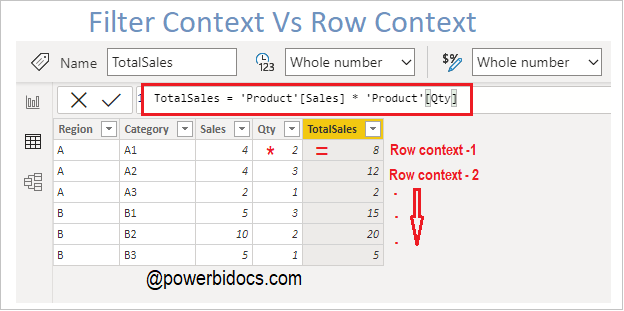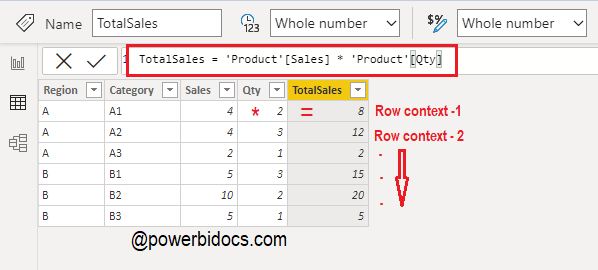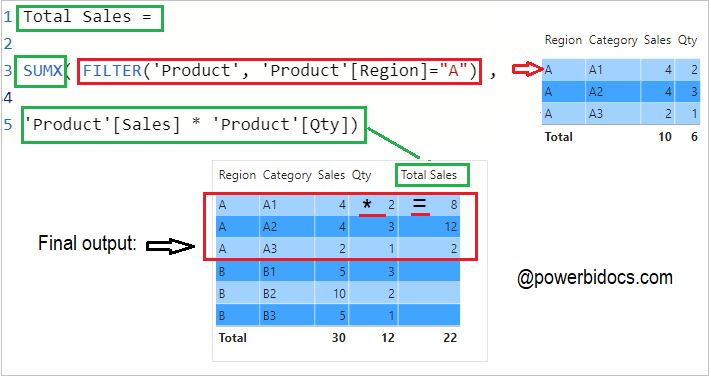Home » Power BI » Filter Context and Row Context in Power BI

# Filter Context and Row Context in Power BIMainly there are two types of evaluation context in DAX and they are : Filter context & Row context.

### Filter Context:

The filter context is the set of filters applied to the data model before the evaluation of a DAX expression starts. Filter Context always exist before a DAX expression been evaluated.

Some initial level filters context applied on Power BI report page in form of:-

1. Slicer
2. Filter through other Visual
3. In Matrix visual based on Row and column data fields
4. Power BI filtersFilter Context in Power Bi

### Row Context:

A Row context is evaluated whenever an expression iterates over a table. Each individual row in a table has its own row context.

A row context exists in either a calculated column or an iterating DAX functions such as SUMX, AVERAGEX, FILTER & ADDCOLUMNS etc.

If you have created a calculated column, the row context consists of the values in each individual row and values in columns that are related to the current row.

Example:-

To calculate the Total Sales the Qty should be multiplied by the Sales of each category, in a new calculated column TotalSales.

As you know calculated column perform row by row calculation( row context), so you can see the result in below screen shot.Row context in Power BI

## Filter Context and Row Context in same DAX formula

Sample dataset as below:-

 Region Category Sales Qty A A1 4 2 A A2 4 3 A A3 2 1 B B1 5 3 B B2 10 2 B B3 5 1

Step-1: Create a measure and write below DAX

```Total Sales =

SUMX( FILTER('Product', 'Product'[Region]="A") ,
'Product'[Sales] * 'Product'[Qty])```

It is filtering the table for each row where Region is “A” and multiplying sales with quantity.

Step-2: Drag new measure into Table visual to see the final output. It will return the value only for Region “A” with specified condition.Context in Power BI

Hope you enjoyed the post. Your valuable feedback, question, or comments about this post are always welcome or you can leave us message on our Contact form , we will revert to you asap.